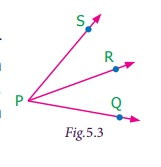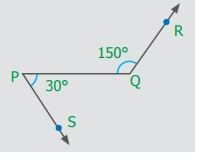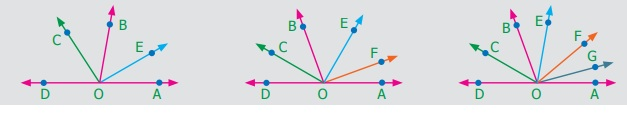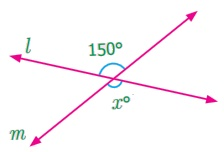Home | | Maths 7th Std | Pair of Angles formed by Intersecting Lines

# Pair of Angles formed by Intersecting Lines

We are going to study related angles such as adjacent angles, linear pair of angles and vertically opposite angles.

Pair of Angles formed by Intersecting Lines

We are going to study related angles such as adjacent angles, linear pair of angles and vertically opposite angles.

The teacher shows a picture of sliced orange with angles marked on it.

Read the conversation between the teacher and students.

Teacher : How many angles are marked on the picture? Can you name them?

Kavin : Three angles are marked on the picture. They are AOC, AOB and BOC

Teacher : Which are the angles seen next to each other?

Thoorigai : Angles such as AOB and BOC are next to each other.

Teacher : How many vertices are there?

Mugil :  There is only one common vertex.Teacher : How many arms are there? Name them.

Amudhan : There are three arms. They are, OB andTeacher : Is there any common arm for AOB and BOC?

Oviya : Yes.is the common arm for AOB and BOC.

Teacher : What can you say about the armsand?

Kavin : They lie on the either side of the common arm.

Teacher : Are the interiors of AOB and BOC overlapping?

Mugil : No. Their interiors are not overlapping.

Teacher : Hence the two angles, AOB and BOC have one common vertex (O), one common arm (), other two arms (and) lie on either side of the common arm and their interiors do not overlap.

Such pair of angles AOB and BOC are called adjacent angles.

So, two angles which have a common vertex and a common arm, whose interiors do not overlap are called adjacent angles.

Now observe the Fig.5.2 in which angles are named 1, 2 and 3.

It can be observed that there are two pairs of adjacent angles such as 1, 2 and 2, 3. Then what about the pair of angles 1 and 3?They are not adjacent because this pair of angles have a common vertex but they do not have a common arm as 2 is in between 1 and 3. Also interiors of 1 and 3 do not overlap. Since the pair of angles does not satisfy one among the three conditions they are not adjacent.

Think

In each of the following figures, observe the pair of angles that are marked as 1 and 2. Do you think that they are adjacent pairs? Justify your answer.Try these

1. Few real life examples depicting adjacent angles are shown below.Can you give three more examples of adjecent angles seen in real life?2. Observe the six angles marked in the picture shown. Write any four pairs of adjacent angles and that are not.adjacent angles A and B, B and C

C and D, D and E

E and F

Aand C

B and D

C and E

Dand F

Aand D

3. Identify the common arm, common vertex of the adjacent angles and shade the interior with two colours in each of the following figures.4. Name the adjacent angles in each of the following figure.(ii) ZWY and YWX

2. Linear pair

Observe the Fig.5.3 QPR and RPS are adjacent angles. It is clear that QPR and RPS together will make QPS which is acute. When QPR and RPS are increased QPS becomes (i) right angle, (ii) obtuse angle, (iii) straight angle and (iv) reflex angle as shown in Fig.5.4.If the resultant angle is a straight angle then the angles are called supplementary angles. The adjacent angles that are supplementary lead us to a pair of angles that lie on straight line (Fig.5.4(iii)). This pair of angles are called linear pair of angles.

Try these

1. Observe the following pictures and find the other angle of linear pair.The other angle (i) 96° (ii) 94° (iii) 21o

Think

Observe the figure. There are two angles namely PQR = 150° and QPS = 30°. Is all this pair of supplementary angles a linear pair? Discuss.Example 5.1 In Fig. 5.5, find AOC.

SolutionAOC = AOB + BOC

= 46° + 51°

= 97°

Example 5.2 If POQ = 23° and POR = 62° then find QOR

SolutionWe know that POR = POQ + QOR

62° = 23° + QOR

Subtracting 23° on both sides

62° – 23° = 23° + QOR – 23°

QOR = 39°

Example 5.3 Which of the following pair of adjacent angles will make a linear pair?

(i) 89°, 91° (ii) 105°, 65° (iii) 117°, 62° (iv) 40°, 140°

Solution

(i) Since 89° + 91° = 180°, this pair will be a linear pair.

(ii) Since 105° + 65° = 170° ≠ 180°, this pair cannot make a linear pair.

(iii) Since 117° + 62° = 179° ≠ 180°, this pair cannot make a linear pair.

(iv) Since 40° + 140° = 180°, this pair will be a linear pair.

Example 5.4 Find the missing angle.Solution

(i) Since the angles are linear pair,

ACD + BCD = 180°

123° + BCD = 180°

Subtracting 123° on both sides

123° + BCD – 123° = 180° – 123°

BCD = 57°

(ii) Since the angles are linear pair, LNO + MNO = 180°

46° + MNO = 180°

Subtracting 46° on both sides

46° + MNO – 46° = 180° – 46°

MNO = 134°

Example 5.5 Two angles are in the ratio 3:2. If they are linear pair, find them.

Solution

Let the angles be 3x and 2x

Since they are linear pair of angles, their sum is 180°.

Therefore, 3x+2x = 180°

5x = 180°

x = 180º / 5

x = 36°

The angles are 3x = 3×36 = 108°

2x = 2×36 = 72°

More on linear pairs

Amudhan asked his teacher what would happen if he drew a ray in between a linear pair of angles? The teacher told him to draw it. Amudhan drew the ray as shown in Fig.5.8.

Teacher asked Amudhan, "what can you say about the angles AOB and BOC?". He said that they are adjacent angles. Also it is true that AOB + BOC = AOC.The teacher also asked about the pair of angles AOC and COD. He replied that they are linear pair. Therefore, their sum is 180° i.e. AOC + COD = 180°.

Combining these two results we get AOB + BOC + COD = 180°.

Thus, the sum of all the angles formed at a point on a straight line is 180°.

Think

What would happen to the angles if we add 3 or 4 or 5 rays on a line as given below?We can learn one more result on linear pairs.

Observe the following Fig.5.9.

AB is a straight line. OC is a ray meeting AB at O.

Here, AOC and BOC are linear pair.

Hence AOC + BOC = 180°Also, OD is another ray meeting AB at O.

Again AOD and BOD are linear pair.

Hence AOD + BOD = 180°

Now, AOC, BOC, AOD and BOD are the angles that are formed at the point O.

We can observe that (AOC + BOC) + (AOD + BOD) = 180° + 180° = 360°.

So, the sum of the angles at a point is 360°.

Think

Can you justify the following statement.

AOB + BOC + COD + DOE + EOF + FOA = 360°?Example 5.6 From Fig.5.10, find the measure of ROS.

Solution

We know that QOR + ROS + SOP = 180°26° + ROS + 32° = 180°

ROS + 58° = 180°

Subtracting 58° on both sides

We get, ROS = 180° – 58° = 122°

Example 5.7 In Fig. 5.11, find the value of x°

Solution

98° + 23° + 76° + x° = 360°197° + x° = 360°

x° = 360° − 197° = 163°

3. Vertically opposite angles

We have already studied about intersecting lines. Observe the Fig.5.12. There are two lines namely l and m which are intersecting at a point O and forming four angles at that point of intersection. They are 1, 2, 3 and 4.Consider any one angle among this say 1. The angles which are adjacent to 1 are 2 and 4, 3 is a non-adjacent angle. Similarly, for the remaining three angles two angles will be adjacent and one angle will be non-adjacent. We can observe that an angle and its non-adjacent angle are just opposite to each other at the point of intersection O (vertex). Such angles which are opposite to each other with reference to the vertex are called vertically opposite angles.

When two lines intersect each other, two pairs of non-adjacent angles formed are called vertically opposite angles.

Try these

1. Four real life examples for vertically opposite angles are given below.Give four more examples for vertically opposite angles in your surrounding.2. In the given figure, two linesandintersect at O. Observe the pair of angles and complete the following table. One is done for you.3. Name the two pairs of vertically  opposite angles.PTS and RTQ

PTR and STQ

4. Find the value of x° in the figure given below.xo = 150o

Activity

On a paper draw two intersecting linesand. Let the two lines intersect at O. Label the two pairs of vertically opposite angles as 1, 2 and 3, 4. Make a trace of angles 2 and 3. Place the traced angle 2 on angle 1. Are they equal? Place the traced angle 3 on angle 4. Are they equal? Continue the same for five different pair of intersecting lines. Record your observations and discuss.

Tags : Geometry | Term 1 Chapter 5 | 7th Maths , 7th Maths : Term 1 Unit 5 : Geometry
Study Material, Lecturing Notes, Assignment, Reference, Wiki description explanation, brief detail
7th Maths : Term 1 Unit 5 : Geometry : Pair of Angles formed by Intersecting Lines | Geometry | Term 1 Chapter 5 | 7th Maths